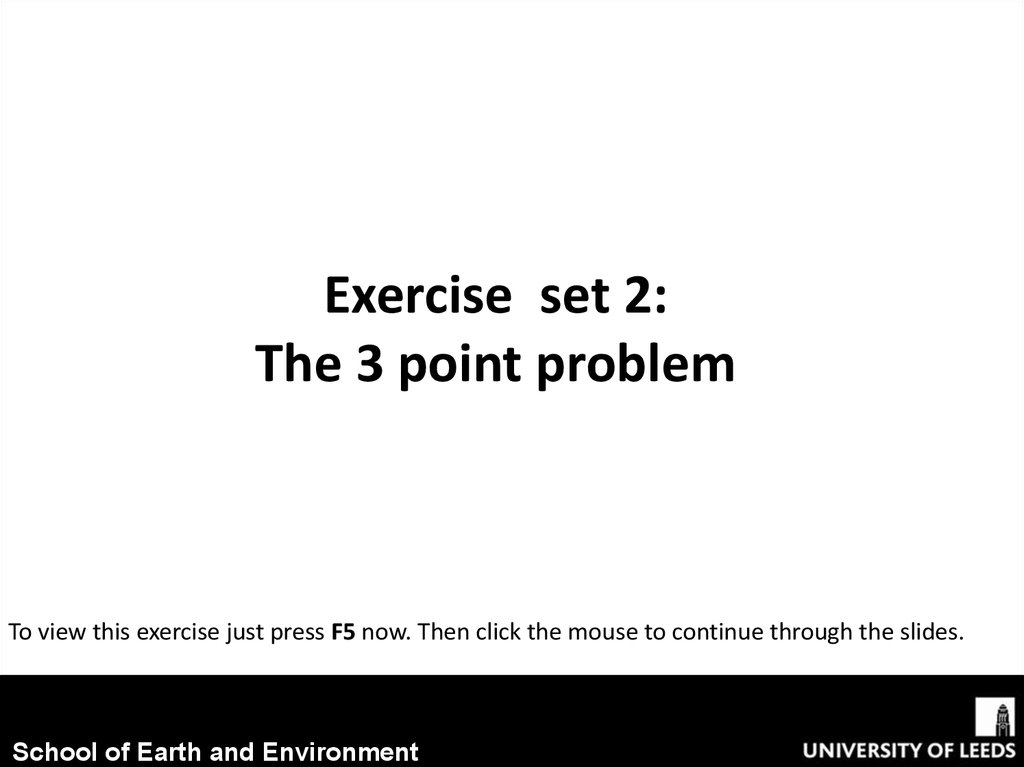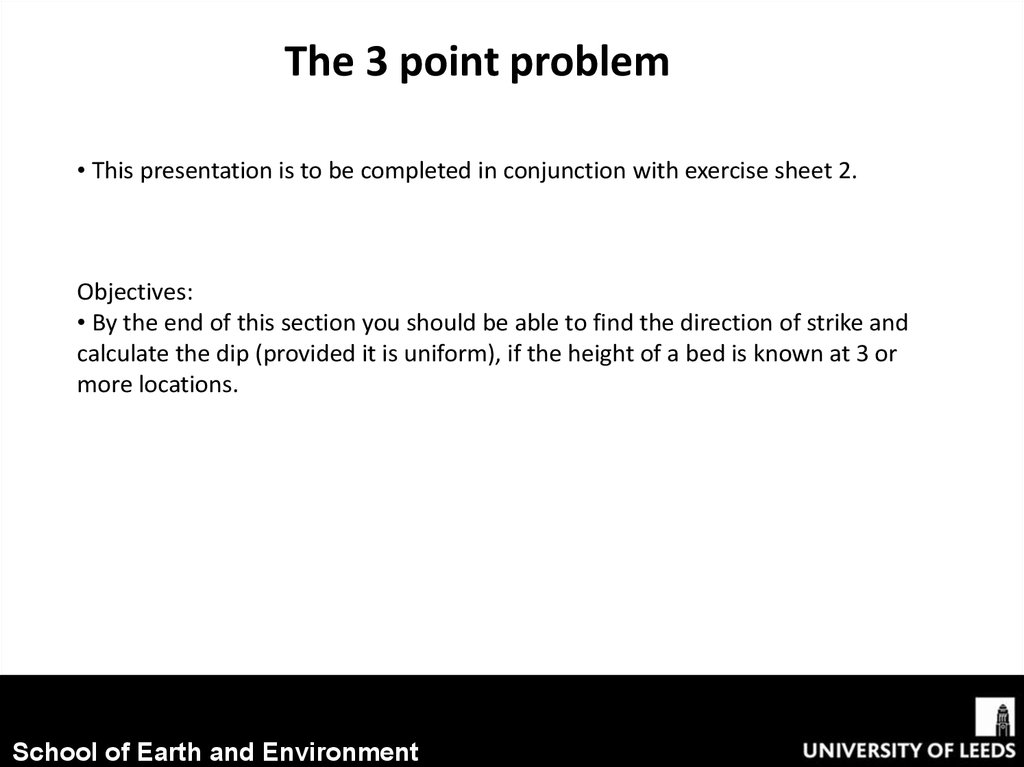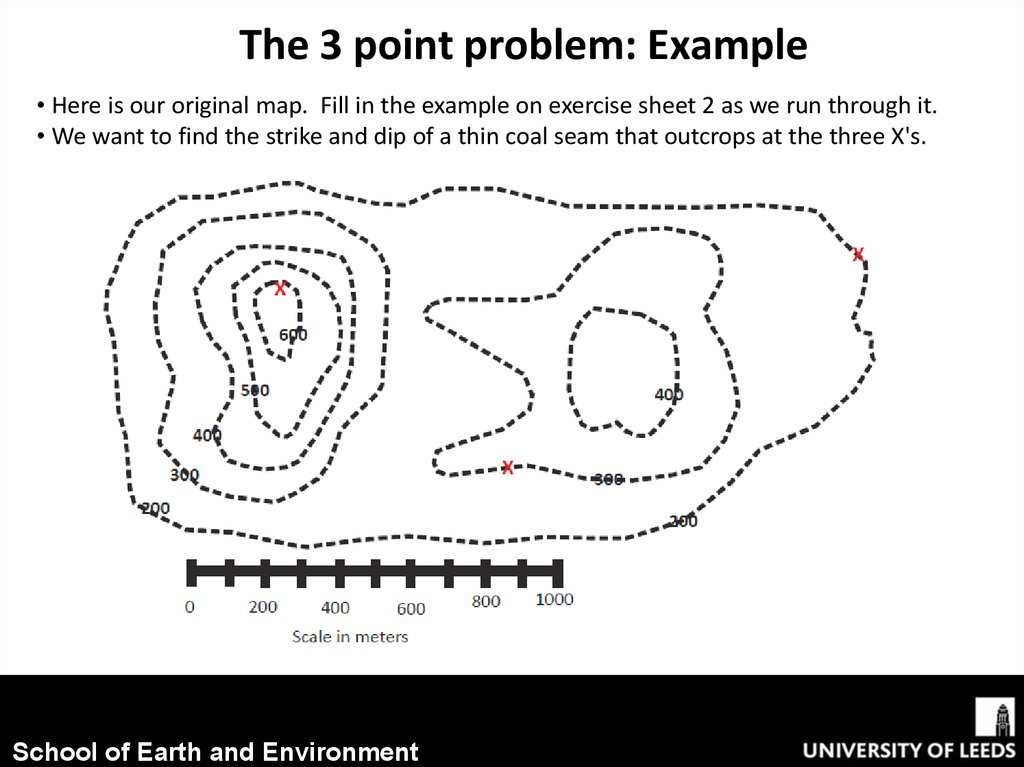1.89M

# The 3 point problem

## 1.

Exercise set 2:
The 3 point problem
To view this exercise just press F5 now. Then click the mouse to continue through the slides.
School of Earth and Environment

## 2.

The 3 point problem
• This presentation is to be completed in conjunction with exercise sheet 2.
Objectives:
• By the end of this section you should be able to find the direction of strike and
calculate the dip (provided it is uniform), if the height of a bed is known at 3 or
more locations.
School of Earth and Environment

## 3.

The 3 point problem: Example
• Here is our original map. Fill in the example on exercise sheet 2 as we run through it.
• We want to find the strike and dip of a thin coal seam that outcrops at the three X's.
School of Earth and Environment

## 4.

The 3 point problem: Example
Step 1:
• Draw a line between the outcrop at the highest elevation and the outcrop at the lowest
elevation.
• If you have two outcrops at the same elevation (which does not apply to this particular
problem), then draw a line between them instead. That is your strike line and you can skip
School of Earth and Environment

## 5.

The 3 point problem: Example
Step 1 continued:
• Measure the map distance of that blue line with your ruler (about 1600m).
• Set up a triangle with the map distance and elevation difference at two ends to calculate
apparent dip.
• The apparent dip tells you that the true dip has to be at least that value, it cannot be
less.
tan(θ) = (400m/1600m)
tan-1(400m/1600m) = θ = 14° = apparent dip
School of Earth and Environment

## 6.

The 3 point problem: Example
Step 2:
• Now, along the blue line you drew, you want to find out where the elevation of the third
point, for this case 300m, would lie (ignore the elevation contours along the blue line).
•Take the difference of elevation between the third point and one of the other given
points.
• We’ll use the outcrop at 200m (300m outcrop – 200m outcrop).
• Now set up another triangle using the apparent dip angle.
School of Earth and Environment

## 7.

The 3 point problem: Example
Step 3:
• Now, measure 401m along the blue line away from the outcrop at 200m (if you used the
outcrop at 600m as one of your other given points in step 2, then adj= 1199m and you
would move 1199m along the blue line from the outcrop at 600m and end up at the same
place) and make a mark (the red circle).
• Now connect that line from the red circle to the third outcrop point – that is your strike
(the green line).
School of Earth and Environment

## 8.

The 3 point problem: Example
Step 4:
• The dip is perpendicular (at a right angle) to the strike line. The dip direction will be in
the general direction of the lower elevation.
• To help understand this, just picture a cross section in your head. With a high point on
the left side and a low point on the right side, the bed would have to be dipping from the
left to the right. (Or be an incredibly thick bed, but we are told it is a thin coal seam).
• So in this case the dip is in the SE direction (the orange line).
School of Earth and Environment

## 9.

The 3 point problem: Example
Step 5:
• To figure out the true dip angle, extend the 300m strike line.
• Connect that strike line to the 200m outcrop so that the line is perpendicular to the
300m line.
•Measure the distance of that purple line with your ruler (about 240m).
School of Earth and Environment

## 10.

The 3 point problem: Example
Step 5 continued:
• Set up another triangle with the elevation difference being 100m (300m strike line –
200m outcrop elevation). The tan of that angle is the true dip.
tan(θ) = (100m/240m)
tan-1(100m/240m) = θ = 23° = true dip
School of Earth and Environment

## 11.

The 3 point problem: Example
Step 5:
• To figure out the outcrop pattern, continue making strike lines with 100m contours.
• Set up another triangle with the elevation 100m and the dip angle 23o to solve for side
• That is how far apart your strike lines should be spaced.
School of Earth and Environment

## 12.

The 3 point problem: Example
Step 5 continued:
• Now draw in your structure contours.
School of Earth and Environment

## 13.

The 3 point problem: Example
Step 5 continued:
• After you’ve drawn in your structure contours (they should be evenly spaced), make a
mark (the yellow dots) every time a structure contour crosses a topographic contour of
the same value.
School of Earth and Environment

## 14.

The 3 point problem: Example
Step 5 continued:
• Now roughly connect up your yellow dots and you have an estimated outcrop pattern!
School of Earth and Environment

## 15.

The 3 point problem: Problem
• Now have a go at the next problem by yourself, by filling in the problem map
on exercise sheet 2.
Questions
• Deduce the strike and dip of the coal seam which is seen to outcrop at points A, B and C.
• Fill in the outcrop pattern.
• At what depth would the coal be encountered in a borehole at D?
School of Earth and Environment

## 16.

Step 1
Scale= 500m/2.5cm = 20m per mm;
Therefore distance from C to A =
107mm= 2140m
tan(θ) = (400m/2140m)
tan-1(400m/2140m) = θ = 10.6°
Apparent dip = 10.6°
School of Earth and Environment

## 17.

Step 2
School of Earth and Environment

## 18.

Step 4
3
School of Earth and Environment

## 19.

Step 5
tan(θ) = (200m/1000m)
tan-1(200m/1000m) = θ = 11.3°
True dip= 11.3°
Then:
School of Earth and Environment

## 20.

Step 5
• Now draw in the structure contours.
• As the length of the opposite of the
triangle was 200m this is the distance
between the contours.
• Therefore halfway between each, add in
the 100m contour intervals.
School of Earth and Environment

## 21.

Step 5
•Now add in the areas the coal
seam will outcrop at.
School of Earth and Environment

## 22.

Step 5
•Now fill in the outcrop pattern
School of Earth and Environment

## 23.

Questions
• Deduce the dip and strike of the coal seam which is seen to outcrop at points A, B
and C.
The actual dip was calculated in step 5 as: 11.3o
The strike is the orientation of the contour lines from North (use a
compass or protractor to measure this, it is always the number less than
180. This is calculated as around: 049o
Therefore the strike/dip can be written as:
049/11 SE
• Fill in the outcrop pattern.
This was done in step 5.
• At what depth would the coal be encountered in a borehole at D?
As location D is on/close to the 400m topographic contour and is also on
the 200m structure contour, the depth that the coal seam would be
encountered in a borehole is:
400m-200m= 200m depth
School of Earth and Environment

## 24.

Summary
• We have now worked through how to find the direction of strike and
calculate the dip (provided it is uniform), if the height of a bed is known at 3 or
more locations, using the 3-point problem.
• If you find this tricky to visualise, there is a 3 dimensional model that can be constructed.
This can be found at the back of the worksheet for exercise 2 and on the following slide.
School of Earth and Environment

## 25.

3 dimensional model of:
School of Earth and Environment